• 设P(Y|X)为线性链条件随机场，则在随机变量X取值为x，的条件下，随机变量Y取值为y的条件概率具有如下形式：其中，上式为线性条件随机场模型的基本形式，表示给定输入序列x，对应序列y预测的条件概率。下面通过一个...
设P(Y|X)为线性链条件随机场，则在随机变量X取值为x，的条件下，随机变量Y取值为y的条件概率具有如下形式：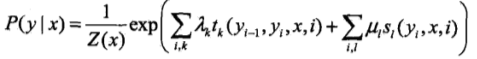其中，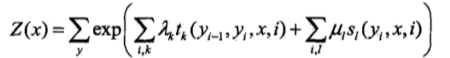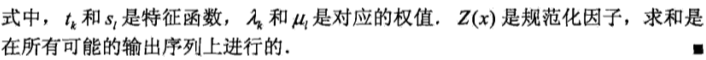上式为线性条件随机场模型的基本形式，表示给定输入序列x，对应序列y预测的条件概率。下面通过一个简单的例子进一步了解;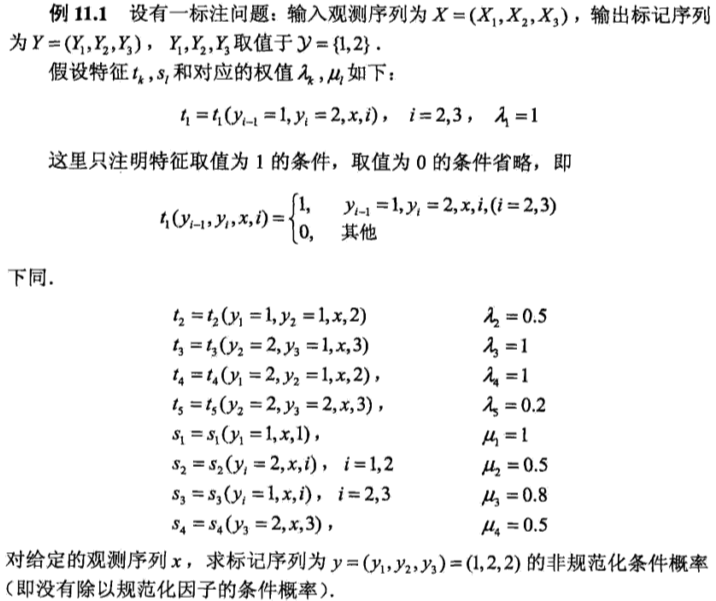解，由上式，线性条件随机场模型为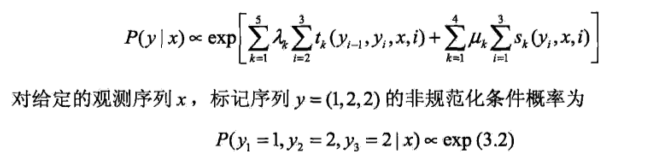展开全文• 条件随机场参数化形式详解
1.条件随机场概念CRF，Conditional Random Field，是给定一组输入随机变量条件下另一组输出随机变量的条件概率分布模式，其特点是假设输出随机变量构成马尔可夫随机场。条件随机场用于不同的预测问题。CRF条件随机场是给定随机变量X时，随机变量Y的马尔可夫随机场。有一种条件随机场是线性链条件随机场（Linear Chain Conditional Random Field）。线性链条件随机场可以用于标注等问题。then，在条件概率P(Y|X)中，Y是输出变量，表示标记序列，X是输入变量，表示需要标注的观测序列。也把标记序列称为状态序列（见隐马尔可夫模型）。2.条件随机场的参数化形式现在有一标注问题：输入观测序列为X=（X1，X2，X3）,输出标记序列为Y=（Y1，Y2，Y3），Y1，Y2，Y3取值于{1，2}.假设特征t<k>，s<l>的对应的权值为λ<k>，μ<l>，公式如下所示：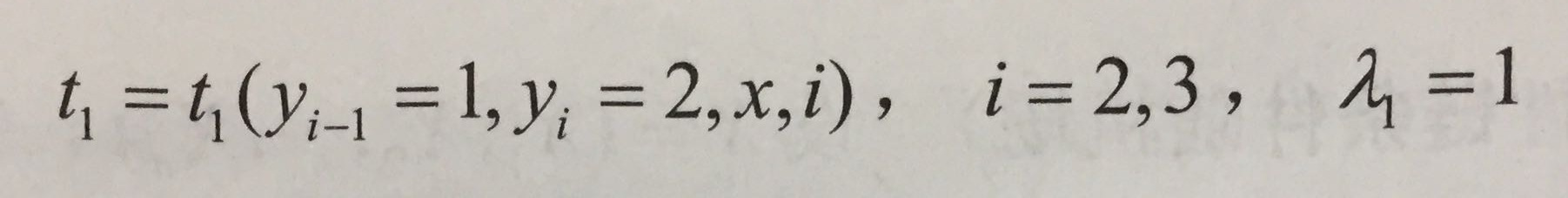这里只注明特征取值为1的条件，取值为0的条件省略，如下：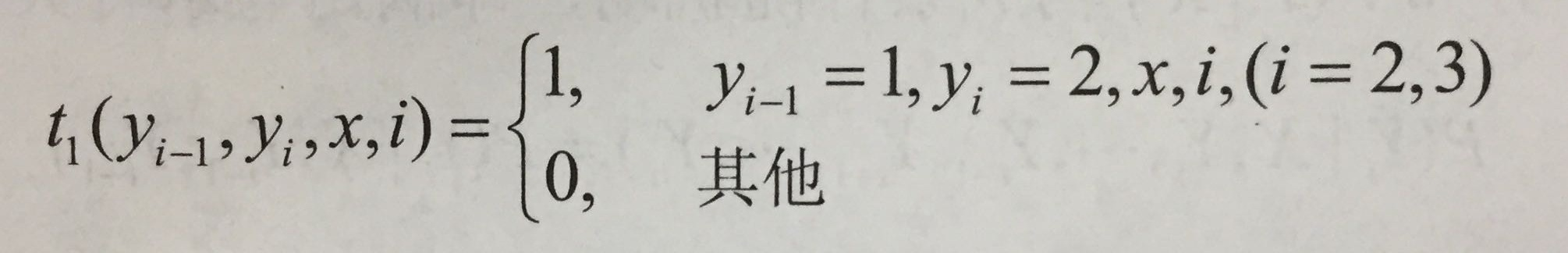下同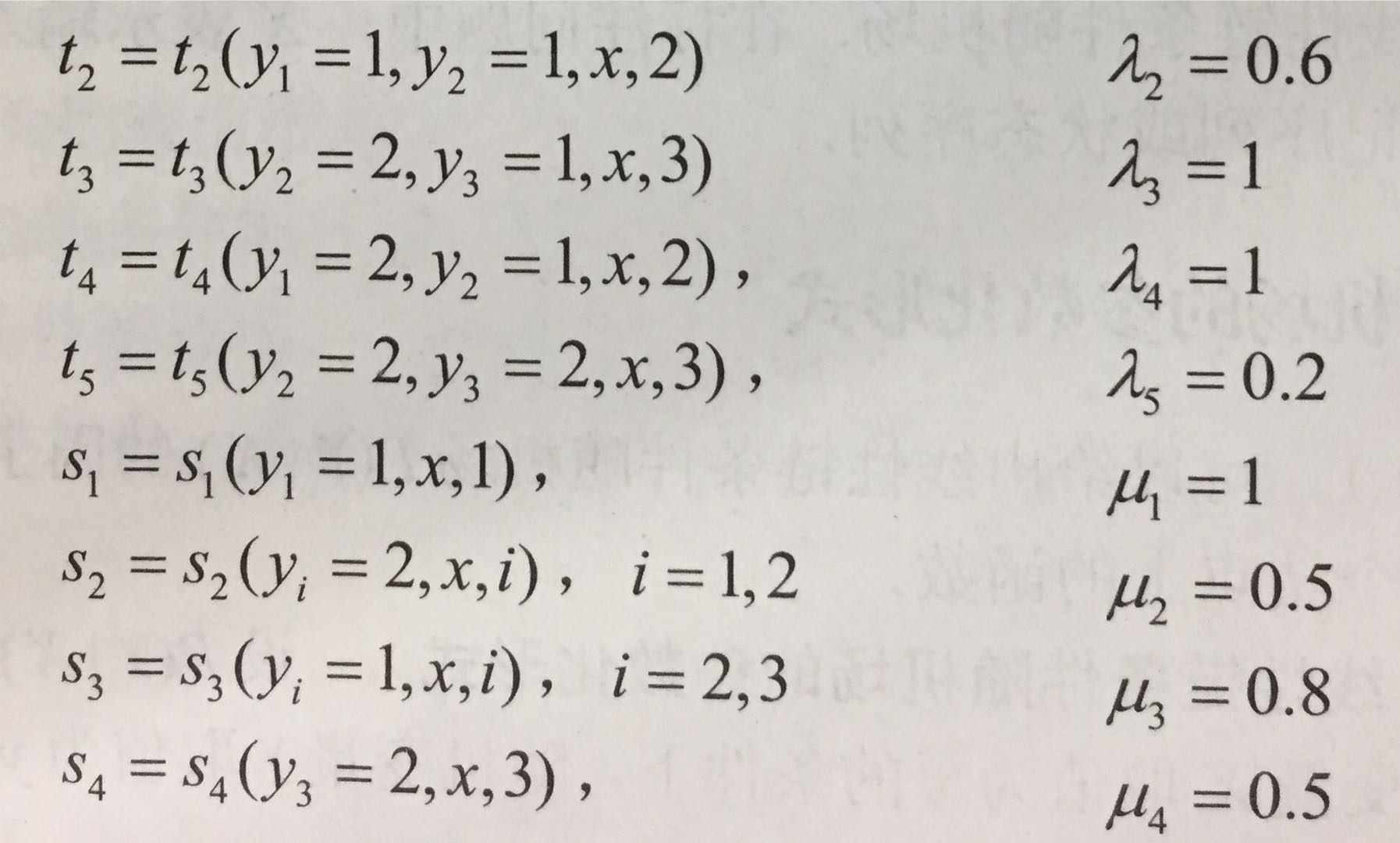说明：t<k>是定义在边上的特征函数，称为转移特征，依赖于当前和前一个位置，s<l>是定义在节点上的特征函数，称为状态特征，依赖于当前位置。这部分内容属于：条件随机场的参数形式的知识。关于条件随机场的参数化形式，详见前面博客：点我。3.参数化形式对应的状态路径图《统计学习方法》中这个示例，作者没有给出这个条件随机场的参数化形式 对应的状态路径图。看着这个参数形式t<k>和s<l>理解其对应的条件随机场，是不太形象的，也不好理解。所以下面人肉给出了此条件随机场的参数化形式对应的状态路径图，看着此图会发现，it's so easy~，有木有，详见下图：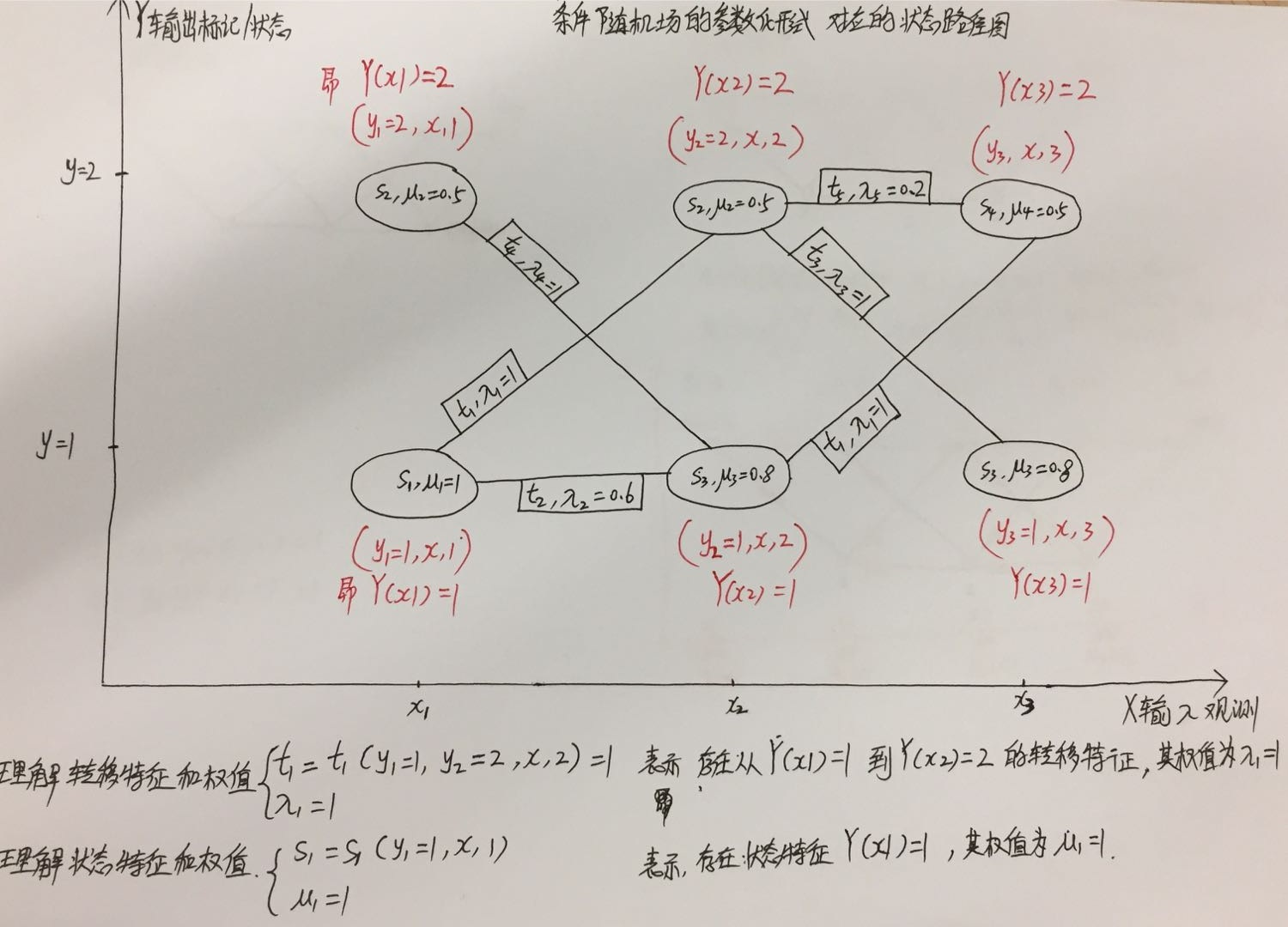上图中给出了对t和s函数的理解，如果仔细看，应该很容易清楚其含义，然后就很容易地画出条件随机场的参数化形式对应的状态路径图~4.参数化形式对应的矩阵表示S矩阵表示定义到节点上的特征函数S(l)，SW矩阵表示每个节点的权值E矩阵表示定义在边上的特征函数T(k)，也称转移特征EW矩阵表示每个转移特征T(k)的权值    S = np.array([[1,1],   #X1:S(Y1=1), S(Y1=2)
[1,1],   #X2:S(Y2=1), S(Y2=2)
[1,1]])  #X3:S(Y3=1), S(Y3=1)
SW = np.array([[1.0, 0.5], #X1:SW(Y1=1), SW(Y1=2)
[0.8, 0.5], #X2:SW(Y2=1), SW(Y2=2)
[0.8, 0.5]])#X3:SW(Y3=1), SW(Y3=1)
E = np.array([[[1, 1],  #Edge:Y1=1--->(Y2=1, Y2=2)
[1, 0]], #Edge:Y1=2--->(Y2=1, Y2=2)
[[0, 1],  #Edge:Y2=1--->(Y3=1, Y3=2)
[1, 1]]])#Edge:Y2=2--->(Y3=1, Y3=2)
EW= np.array([[[0.6, 1],  #EdgeW:Y1=1--->(Y2=1, Y2=2)
[1, 0.0]], #EdgeW:Y1=2--->(Y2=1, Y2=2)
[[0.0, 1],  #EdgeW:Y2=1--->(Y3=1, Y3=2)
[1, 0.2]]])#EdgeW:Y2=2--->(Y3=1, Y3=2)也就是说上面W,SW,E,EW这四个矩阵就表示了上面参数化形式表示的条件随机场，参数化形式表示的条件随机场和矩阵形式表示的条件随机场，以及状态路径图表示的条件随机场都是等价的。到此，条件随机场的参数化形式解释结束了。（end）
展开全文• 第一部分给出概率图模型的定义与性质，以及对它意义重大的因子分解定理；第二部分给出条件随机场本质是概率图模型这一定义，而后针对使用最多的线性链条件随机场给出概率计算以及参数学习算法。

第一部分给出概率图模型的定义与性质，以及对它意义重大的因子分解定理；第二部分给出条件随机场本质是概率图模型这一定义，而后针对使用最多的线性链条件随机场给出概率计算以及参数学习算法。

文章目录概率无向图.条件随机场.条件随机场参数化.条件随机场向量化.条件随机场矩阵形式.
概率无向图.

概率图模型 Probabilistic Graphical Model 是借助图表示的概率分布，现假设有联合概率分布 $P(Y),Y\in\mathcal Y$ 是一组随机变量，我们以无向图 $G=(V,E)$ 来表示这一概率分布，其中顶点 $v\in V$ 表示一个随机变量 $Y_v$，边 $e\in E$ 表示随机变量之间的概率依赖关系。
概率图模型需要满足马尔可夫性，并且在图表示中，马尔可夫性的表现形式可以是如下三种，不难验证它们本质上是等价的。
【成对马尔可夫性 Pairwise】对于无向图 $G$ 中任意两个没有边连接的顶点 $u,v$，它们对应的随机变量分别为 $Y_u,Y_v$，其余所有顶点集合记为 $O$，对应的随机变量组为 $Y_O$，那么在给定随机变量组 $Y_O$ 的条件下，$Y_u$ 和 $Y_v$ 条件独立：$P(Y_u,Y_v|Y_O)=P(Y_u|Y_O)\cdot P(Y_v|Y_O)$
【局部马尔可夫性 Local】设 $v$ 是无向图 $G$ 中的任意一个顶点，$W$ 为所有与 $v$ 有边相连的顶点集合，$O$ 是除 $v,W$ 以外的所有顶点集合，它们对应的随机变量、随机变量组分别为 $Y_v,Y_W,Y_O$，那么在给定随机变量组 $Y_W$ 的条件下，$Y_v$ 和 $Y_O$ 条件独立：$P(Y_v,Y_O|Y_W)=P(Y_v|Y_W)\cdot P(Y_O|Y_W)$并且根据贝叶斯公式，当 $P(Y_O|Y_W)>0$ 时我们有：$P(Y_v|Y_W)=\frac{P(Y_v,Y_O|Y_W)}{P(Y_O|Y_W)}=P(Y_v|Y_W,Y_O)$直观上来看，给定条件 $Y_W$ 时 $Y_v$ 的概率分布和给定条件为 $Y_W\wedge Y_O$ 时 $Y_v$ 的概率分布相同，我们可以简单地认为 $Y_O$ 并不会影响 $Y_v$ 的概率分布，但严格上还需要注意这里是条件独立，与二者独立还是有区别的：$P(Y_v,Y_O)=P(Y_v)\cdot P(Y_O)$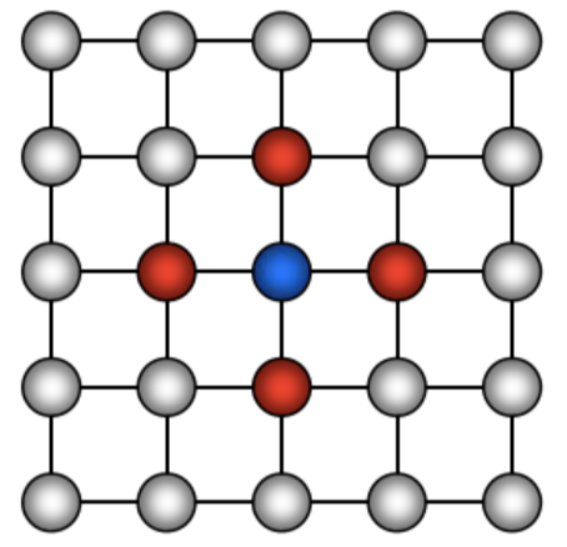上图中蓝色点为 $v$，红色点集合为 $W$，其余所有灰色节点为 $O.$
【全局马尔可夫性 Global】设顶点集合 $A,B$ 是无向图 $G$ 中被顶点集合 $C$ 分开的任意顶点集合，它们对应的随机变量组分别为 $Y_A,Y_B,Y_C$，那么在给定 $Y_C$ 的条件下，$Y_A$ 和 $Y_B$ 条件独立：$P(Y_A,Y_B|Y_C)=P(Y_A|Y_C)\cdot P(Y_B|Y_C)$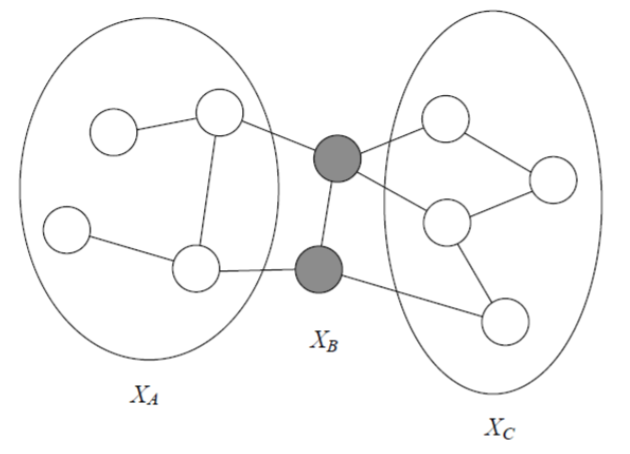上图中 $X_A,X_C$ 在给定条件 $X_B$ 下是条件独立的。
【Defn】对于联合概率分布 $P(Y)$，由无向图 $G=(V,E)$ 表示，其中顶点表示随机变量，边表示随机变量之间的依赖关系。如果联合概率分布 $P(Y)$ 满足上述三种马尔可夫性的一种，那么就称此联合概率分布为 概率无向图模型PGM 或 马尔可夫随机场MRF.
对于给定的概率无向图模型，我们更关心的是如何求出其表示的联合概率分布，因子分解定理的意义就是 —— 整体的联合概率分布可以表示为若干个子联合概率分布的乘积。

在给出因子分解定理之前，我们回忆一下图论中团与极大团的定义，无向图 $G$ 中任意两个顶点之间均有边相连的顶点集合称为团，显然团与其确定的边构成完全图；而图 $G$ 的极大团则意味着该团中不能再加入任何一个 $G$ 中顶点使其成为一个更大的团，称之为极大团maximal clique.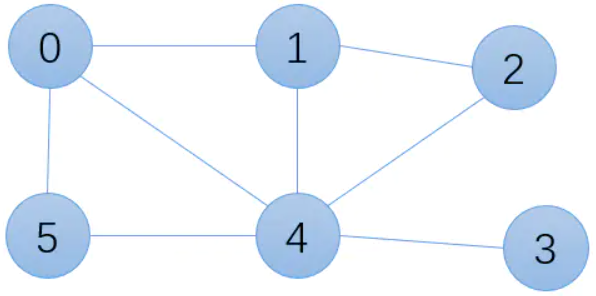对于上图而言，下面的顶点集都是它的团：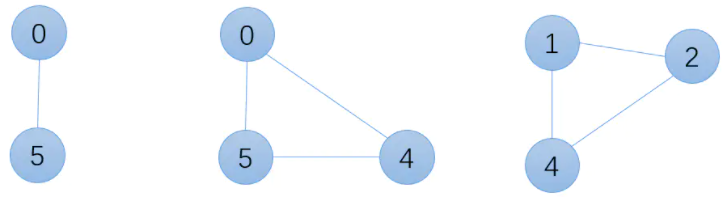- 但显然 $\{0,5\}$ 中可以加入顶点 $4$ 使之成为更大的团，因此 $\{0,5\}$ 不是极大团，但显然上述图中并不存在完全图 $K_4$，因此我们可以断言 $\{0,4,5\},\{1,2,4\}$ 均为极大团。上图的全部极大团如下：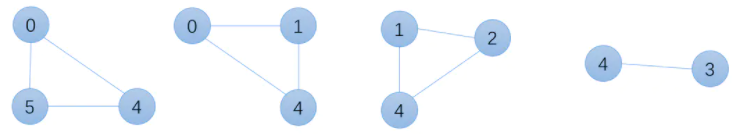注意到 $\{3,4\}$ 是顶点数为 $2$ 的极大团，向其中加入任何一个顶点都会使其不再是团，另外还有最大团的概念，即基数最大的极大团，也就是上图中的前三个极大团，它们基数相同，均为 $3.$

将概率无向图的联合概率分布表示为其极大团上的随机变量组的函数乘积形式，称为概率无向图的因子分解Factorization. 给定概率无向图 $G=(V,E)$，$C$ 表示图 $G$ 的极大团，$Y_C$ 表示对应的随机变量组，那么联合概率分布 $P(Y)$ 可以表示如下：$P(Y)=\frac1Z\prod_C\Psi_C(Y_C)\tag{1}$其中 $\Psi_C$ 被称为势函数，要求是严格正的，常见形式为指数函数：$\Psi_C(Y_C)=\exp\Big(-E[Y_C]\Big)$$Z$ 是规范化因子，保证 $P(Y)$ 构成概率分布，由下式给出：$Z=\sum_Y\prod_C\Psi_C(Y_C)$ 这里对 $Y$ 求和的意义并不直观，个人理解是对所有可能的随机变量取值求和，这样可以和后续条件随机场中的物理意义相一致。
上述定理即为因子分解定理，也由于提出者的名字被称为 Hammersley-Clifford 定理，它的意义就是概率无向图所表示的联合概率分布可以被写作其所有极大团对应随机变量组势函数的乘积形式，注意乘积的对象是所有极大团。

条件随机场.

条件随机场 Conditional Random Field是给定随机变量 $X$ 条件下随机变量 $Y$ 的马尔可夫随机场(概率无向图)，本篇主要讲述的是定义在线性链上的特殊条件随机场，称为线性链条件随机场，多用于标注问题。条件随机场的模型形式为 $P(Y|X)$，其中 $Y$ 是输出变量，可以视作标注序列；$X$ 是输入变量，是待标注的观测序列。
类比隐马尔科夫模型中观测序列和状态序列的概念，我们也将 $Y$ 称作状态序列，在学习时我们利用训练数据集，进行极大似然估计(正则化极大似然估计)来得到条件概率模型 $\hat P(Y|X)$；预测时对于给定的观测序列 $x$，输出 $\argmax_y\hat P(y|x)$ 即可。
条件随机场的形式化定义如下：设 $X,Y$ 是随机变量，$P(Y|X)$ 是在给定 $X$ 条件下 $Y$ 的条件概率分布，如果 $Y$ 构成一个马尔可夫随机场(由无向图 $G$ 表示)，并且下式：$P(Y_v|X,Y_u,u\neq v)=P(Y_v|X,Y_u,u\sim v)$对于任意的 $v$ 都成立，则称条件概率分布 $P(Y|X)$ 为条件随机场。
其中 $u\neq v$ 表示除顶点 $v$ 以外的所有顶点，$u\sim v$ 表示和 $v$ 有边连接的所有顶点，$Y_v,Y_u$ 表示与之对应的随机变量，上式直观来看说明了只有那些 $v$ 直接相连的顶点才存在随机变量之间的依赖关系。

【线性链条件随机场】设 $X=\{X_1,X_2,\cdots,X_n\},Y=\{Y_1,Y_2,\cdots,Y_n\}$ 均为线性链表示的随机变量序列，若在给定随机变量序列 $X$ 的条件下，随机变量序列 $Y$ 的条件概率分布构成马尔可夫随机场，即满足如下线性链情况下的马尔可夫性：$P(Y_i|X,Y_1,Y_2,\cdots,Y_{i-1},Y_{i+1},\cdots,Y_n)=P(Y_i|X,Y_{i-1},Y_{i+1})$那么条件概率分布 $P(Y|X)$ 就成为线性链条件随机场，注意上式在 $i=1,i=n$ 时需要特殊考虑。

条件随机场参数化.

以下所有的讨论都是针对线性链条件随机场而言的，简记为条件随机场。其直观形式如下图所示：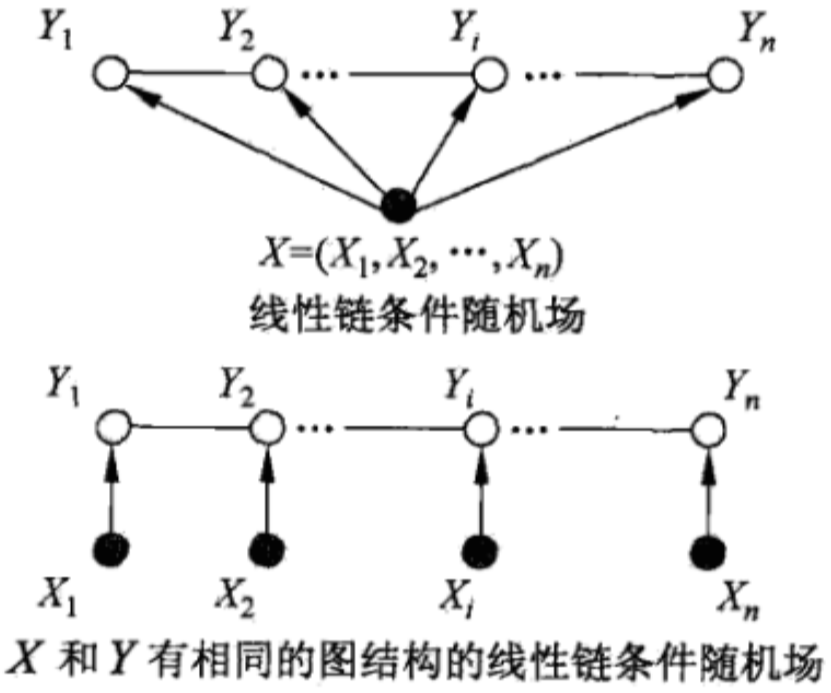我们进行形式化推导时一般以第一种 —— 将 $X$ 表示为单个顶点为辅助理解图。
对上述线性链条件随机场应用因子分解定理，即条件概率分布 $P(Y|X),Y=\{Y_1,Y_2,\cdots,Y_n\}$ 可以被表示为因子乘积的形式 ，其中每个因子是定义在极大团上的势函数，并且借助上图我们可以发现，对于以 $\{Y_1,Y_2,\cdots,Y_n\}$ 为顶点的概率图模型而言，其极大团就是相邻的两个顶点，我们定义如下形式的势函数：$\ln\Psi_C(Y_C)=\ln\Psi_C(y_{i-1},y_i)=\sum_k\lambda_k\cdot t_k(y_{i-1},y_i,x,i)+\sum_{l}\mu_l\cdot s_l(y_i,x,i)$因此对于 $(1)$ 式而言，我们有：$P(Y|X)=\frac1{Z(x)}\prod_C\Psi_C(Y_C)=\frac1{Z(x)}\exp\Big(\sum_i\ln\Psi_C(y_{i-1},y_i)\Big)$因此我们得到线性链条件随机场的参数化形式：$P(Y|X)=\frac1{Z(x)}\exp\Big(\sum_i\sum_k\lambda_k\cdot t_k(y_{i-1},y_i,x,i)+\sum_i\sum_l\mu_l\cdot s_l(y_i,x,i)\Big)\tag{2}$
其中 $t_k,s_l$ 被称为特征函数，$\lambda_k,\mu_l$ 是对应的权值，而规范化因子 $Z(x)$ 的计算方法如下：$Z(x)=\sum_y\exp\Big(\sum_i\ln\Psi_C(y_{i-1},y_i)\Big)$其求和的对象是所有可能的输出序列。
$t_k(\cdot)$ 是定义在概率图中边上的特征函数，称为转移特征，依赖于当前位置和前一位置，同一条边上可以有不同的边特征函数 $t_{k1}(\cdot),t_{k2}(\cdot)$；对应的，$s_l(\cdot)$ 是定义在概率图中顶点上的特征函数，称为状态特征，依赖于当前位置。可以归纳发现，特征函数 $t_k,s_l$ 都依赖于局部位置，是局部特征函数，通常定义为 $\{0,1\}$ 二值函数，满足特征条件时取 $1$，不满足时取 $0.$ 线性链条件随机场能够完全由特征函数和对应的权值确定。

【例】场景为标注问题，输入观测序列为 $X=\{X_1,X_2,X_3\}$，输出标记序列为 $Y=\{Y_1,Y_2,Y_3\},Y_i\in\{0,1\}.$
对于转移特征函数，我们定义如下：$t_1=t_1(y_1=1,y_2=2,x,2),~\lambda_1=1$$t_2=t_2(y_2=1,y_3=2,x,3),~\lambda_2=1$$t_3=t_3(y_1=1,y_2=1,x,2),~\lambda_3=0.6$$t_4=t_4(y_2=2,y_3=1,x,3),~\lambda_4=1$$t_5=t_5(y_1=2,y_2=1,x,2),~\lambda_5=1$$t_6=t_6(y_2=2,y_3=2,x,3),~\lambda_6=0.2$可以发现在同一条边上存在不同的转移特征函数，它们对应的权值由 $\lambda_i$ 表示。
对于状态特征函数，定义如下：$s_1=s_1(y_1=1,x,1),~\mu_1=1$$s_2=s_2(y_1=2,x,1),~\mu_2=0.5$$s_3=s_3(y_2=2,x,2),~\mu_3=0.5$$s_4=s_4(y_2=1,x,2),~\mu_4=0.8$$s_5=s_5(y_3=1,x,3),~\mu_5=0.8$$s_6=s_6(y_3=2,x,3),~\mu_6=0.5$可以发现对于相同的顶点，也存在不同的状态特征函数，其权值由 $\mu_i$ 表示。
对于给定的观测序列 $x$，如果我们要求输出状态序列为 $y=(1,2,2)$ 的非规范化概率，即没有除以 $Z(x)$ 的值，计算方法为：$P\Big(y=(1,2,2)|x\Big)\propto\exp\Big[\sum_{k=1}^6\lambda_k\sum_{i=2}^3t_k(y_{i-1},y_i,x,i)+\sum_{l=1}^6\mu_l\sum_{i=1}^3s_l(y_i,x,i)\Big]$代入实际数值计算后得到指数部分为：$\lambda_1\cdot t_1(1,2,x,2)+\lambda_6\cdot t_6(2,2,x,3)+\mu_1\cdot s_1(1,x,1)+\mu_3\cdot s_3(2,x,2)+\mu_6\cdot s_6(2,x,3)=3.2$因此：$P\Big(y=(1,2,2)|x\Big)\propto\exp(3.2)$

条件随机场向量化.

在上一部分参数化的基础上，对条件随机场进行向量化。声明：这一步骤不涉及本质问题，仅仅是数学形式上的等价推导。
考察 $(2)$ 式我们发现，每个特征函数 $t_k,s_l$ 都对于位置参数 $i$ 进行了求和操作，我们尝试先进行局部特征函数对于位置参数的求和，得到全局特征函数，具体操作如下：我们有 $K_1$ 个转移特征函数，$K_2$ 个状态特征函数，将他们统一起来，即令 $K=K_1+K_2$，并定义：
f_k(y_{i-1},y_i,x,i)=\left\{ \begin{aligned} &t_k(y_{i-1},y_i,x,i),k=1,2,\cdots,K_1\\ &s_l(y_i,x,i),k=K_1+l,l=1,2,\cdots,K_2 \end{aligned} \right.相应地权值定义如下：w_k=\left\{ \begin{aligned} &\lambda_k,k=1,2,\cdots,K_1\\ &\mu_l,k=K_1+l,l=1,2,\cdots,K_2 \end{aligned} \right.
将函数 $f_k$ 对位置参数 $i$ 求和，得到：$f_k(y,x)=\sum_{i=1}^nf_k(y_{i-1},y_i,x,i),~k=1,2,\cdots,K$于是 $(2)$ 式可以改写为：$P(y|x)=\frac1{Z(x)}\exp\Big(\sum_{k=1}^Kw_k\cdot f_k(y,x)\Big)$$Z(x)=\sum_y\exp\Big(\sum_{k=1}^Kw_k\cdot f_k(y,x)\Big)$
进一步向量化，令：$w=(w_1,w_2,\cdots,w_K)^T$$F(y,x)=\Big(f_1(y,x),f_2(y,x),\cdots,f_K(y,x)\Big)^T$那么上式可以进一步简化为：$P(y|x)=\frac1{Z(x)}\exp\Big(w\cdot F(y,x)\Big)$$Z(x)=\sum_y\exp\Big(w\cdot F(y,x)\Big)$

条件随机场矩阵形式.

矩阵形式不同于前面的直接参数化 $(2)$ 式，基于条件随机场的矩阵形式能够快速计算出给定输入序列 $x$ 条件下某个状态序列 $y$ 的条件概率 $P(y|x).$ 在矩阵形式中我们对于每个状态序列引入一个特殊的起点 $y_0$ 和特殊的终点 $y_{n+1}.$
对于从 $i=1,2,\cdots,n+1$ 范围内的 $y_{i-1},y_i$ 而言，我们假设它们的取值集合基数为 $m$，那么我们可以定义一个 $m$ 阶的矩阵 $M_i(x)$，其中的元素为：$M_i(x){[y_{i-1},y_i]}=\exp\Big(\sum_{k=1}^Kw_k\cdot f_k(y_{i-1},y_i,x,i)\Big)$
基于这样的定义，给定观测序列 $x$，某个状态序列 $y$ 的非规范化概率就可以通过 $n+1$ 个矩阵 $M_i(x)$ 中对应位置元素的乘积来得到，即：$P(y|x)\propto\prod_{i=1}^{n+1}M_i(x)[y_{i-1},y_i]=M_1(x)[y_0,y_1]\cdot M_2(x)[y_1,y_2]\cdots M_{n+1}(x)[y_n,y_{n+1}]$对上式进行规范化得到：$P(y|x)=\frac1{Z(x)}\prod_{i=1}^{n+1}M_i(x)[y_{i-1},y_i]$$Z(x)=\Big(\prod_{i=1}^{n+1}M_i(x)\Big)[y_0,y_{n+1}]$
在我们的假设中，$y_0$ 和 $y_{n+1}$ 分别是起始与终止状态，而 $Z(x)$ 是所有以 $y_0$ 为起始状态，$y_{n+1}$ 为终止状态的路径 $y_1y_2\cdots y_n$ 的非规范化概率之和。

出于前后统一，并起到验证作用，我们对条件随机场参数化中给出的例子，采用矩阵形式进行计算，矩阵的计算我们选取三个矩阵作为示例详细计算，一头一尾以及中间的某个矩阵：$M_1(x),M_4(x),M_2(x)$，并且本例中我们假设 $y_0=1,y_4=1.$
对于 $M_1(x)$ 而言，其元素的计算式如下：$M_1(x)[y_0,y_1]=\exp\Big(\sum_{k=1}^{12}w_k\cdot f_k(y_0,y_1,x,1)\Big)$由于前面假定了 $y_0=1$，所以我们只需要计算元素 $[1,1],[1,2]$，其计算式分别为：$M_1(x)[1,1]=\exp\Big(\sum_{k=1}^{12}w_k\cdot f_k(1,1,x,1)\Big)=\exp(1)$$M_1(x)[1,2]=\exp\Big(\sum_{k=1}^{12}w_k\cdot f_k(1,2,x,1)\Big)=\exp(0.5)$因此我们不难得到矩阵 $M_1(x)$ 的数值：$M_1(x)=\left[ \begin{matrix} \exp(1) & \exp(0.5) \\ 0 & 0 \end{matrix} \right]$
对于 $M_2(x)$ 而言，其元素 $[1,1],[1,2],[2,1],[2,2]$ 都是需要我们计算的，其计算式分别为：$M_2(x)[1,1]=\exp\Big(\sum_{k=1}^{12}w_k\cdot f_k(1,1,x,2)\Big)=\exp(1.4)$$M_2(x)[1,2]=\exp\Big(\sum_{k=1}^{12}w_k\cdot f_k(1,2,x,2)\Big)=\exp(1.5)$$M_2(x)[2,1]=\exp\Big(\sum_{k=1}^{12}w_k\cdot f_k(2,1,x,2)\Big)=\exp(1.8)$$M_2(x)[2,2]=\exp\Big(\sum_{k=1}^{12}w_k\cdot f_k(2,2,x,2)\Big)=\exp(0.5)$因此 $M_2(x)$ 的数值如下：$M_2(x)=\left[ \begin{matrix} \exp(1.4) & \exp(1.5) \\ \exp(1.8) & \exp(0.5) \end{matrix} \right]$
对于 $M_3(x)$ 而言，其元素 $[1,1],[1,2],[2,1],[2,2]$ 都是需要我们计算的，其计算式分别为：$M_3(x)[1,1]=\exp\Big(\sum_{k=1}^{12}w_k\cdot f_k(1,1,x,3)\Big)=\exp(0.8)$$M_3(x)[1,2]=\exp\Big(\sum_{k=1}^{12}w_k\cdot f_k(1,2,x,3)\Big)=\exp(1.5)$$M_3(x)[2,1]=\exp\Big(\sum_{k=1}^{12}w_k\cdot f_k(2,1,x,3)\Big)=\exp(1.8)$$M_3(x)[2,2]=\exp\Big(\sum_{k=1}^{12}w_k\cdot f_k(2,2,x,3)\Big)=\exp(0.7)$因此 $M_3(x)$ 的数值如下：$M_3(x)=\left[ \begin{matrix} \exp(0.8) & \exp(1.5) \\ \exp(1.8) & \exp(0.7) \end{matrix} \right]$
对于 $M_4(x)$ 而言，由于假设了 $y_4=1$，因此我们仅需要计算 $[1,1],[2,1]$，其计算式结果均为 $\exp(0)=1$，因为特征函数中并没有 $i=4$ 的存在，所以其数值如下：$M_4(x)=\left[ \begin{matrix} 1 & 0 \\ 1 & 0 \end{matrix} \right]$
写到这里突然发现，上面每个计算式最终的等号都忘了取自然指数，但不影响计算非规范化概率，我们仍然求状态序列 $y=(1,2,2)$ 的条件概率，可以得到：$P\Big(y=(1,2,2)|x\Big)\propto\exp(1+1.5+0.7+0)=\exp(3.2)$并且归一化因子 $Z(x)$ 的计算式如下：$Z(x)=\Big(\prod_{i=1}^4M_i(x)\Big)[1,1]$表示了所有以 $y_0=1,y_4=1$ 为始、终状态的状态序列 $y_1y_2y_3$ 的非规范化概率之和。

在下篇中将会介绍线性链条件随机场的概率计算 $P(Y_i=y_i|x),P(Y_{i-1}=y_{i-1},Y_i=y_i|x)$，这里用到的算法是和HMM中类似的前向-后向算法；线性链条件随机场的参数学习方法，我们可以看到这里的问题情境是监督学习，因此可以基于极大似然估计(以及带正则化的极大似然估计)来实现参数学习，具体的实现方法有改进迭代尺度法、梯度下降法以及拟牛顿法；线性链条件随机场的预测算法，这一问题中又用到了曾经在HMM中介绍过的维特比算法，可以前往《隐马尔科夫模型》中参考更多细节。


展开全文• 文章目录概率无向图模型模型定义概率无向图模型:概率无向图模型的因子分解条件随机场的模型表示linear-chain 条件随机场CRF 的定义CRF 的参数化形式CRF 的简化形式条件随机场的矩阵形式条件随机场的概率计算问题前向...
文章目录概率无向图模型模型定义概率无向图模型:概率无向图模型的因子分解条件随机场的模型表示linear-chain 条件随机场CRF 的定义CRF 的参数化形式CRF 的简化形式条件随机场的矩阵形式条件随机场的概率计算问题前向-后向算法概率计算期望值的计算条件随机场的训练L-BFGS算法条件随机场的预测算法
条件随机场（conditional random field，以下简称CRF） 是给定一组输入随机变量条件下另一组输出随机变量的条件概率分布模型，其特点是假设输出随机变量构成马尔可夫随机场（HMM 是状态序列的 Markov Chain）。CRF 可以用于不同的预测问题，在 Machine Learning 领域里 CRF 一般用作处理标注问题。常用的就是线性链（linear-chain) 条件随机场了，这时，问题变成了由输入序列对输出序列预测的判别模型，形式为对数线性模型，其学习方法通常是极大似然估计或正则化的极大似然估计。
概率无向图模型
概率无向图模型又称为马尔可夫随机场（Markov random field)，是一个可以由无向图表示的联合概率分布。
模型定义
图是由结点及连接结点的边组成的集合。结点和边分别记作 v 和 e ，结点和边的集合分别记作 V 和 E ，图记作 G=(V,E) ，无向图是指边没有方向的图。概率图模型（PGM） 是由图表示的概率分布。设有联合概率分布 P(Y) ，$Y \in \mathcal{Y}$ 是一组随机变量。由无向图 G 表示概率分布，即在图 G 中，结点 v∈V 表示一个随机变量 $Y_v$， $Y = Y_v|_{v \in V}$；边 e∈E 表示随机变量之间的概率依赖关系。
给定一个联合概率分布 P(Y) 和表示它的无向图 G。首先定义无向图表示的随机变量之间存在的成对马尔可夫性局、部马尔可夫性和全局马尔可夫性。分别介绍一下三个概念：
成对马尔可夫性：设 u 和 v 是无向图G中任意两个没有边连接的结点，结点u和v分别对应随机变量 Yu 和 Yv。其他所有结点为 O（集合），对应的随机变量组是 YO。成对马尔可夫性是指给定随机变量组 YO 的条件下随机变量 Yu 和 Yv 是条件独立的，其实意思就是说没有直连边的任意两个节点是独立的，即
$P(Y_v,Y_O |Y_W) = P(Y_v|Y_W)P(Y_O|Y_W)$
局部马尔可夫性：设 v \in V 是无向图 G 中任意一个结点，W 是与 v 有边连接的所有结点，O 是 v,W 以外的其他所有结点。v 表示的随机变量是 Yv ,W 表示的随机变量组是 Y_w，O 表示的随机变量组是 Y_O。局部马尔可夫性是指在给定随机变量组 Y_W 的条件下随机变量 v 与随机变量组  Y_O 是独立的，即
$P(Y_v,Y_O |Y_W) = P(Y_v|Y_W)P(Y_O|Y_W)$
在 $P(Y_O|Y_W) >0$ 时，等价地
$p(Y_v |Y_W) = P(Y_v|Y_W,Y_O)$
下图表示了局部马尔可夫性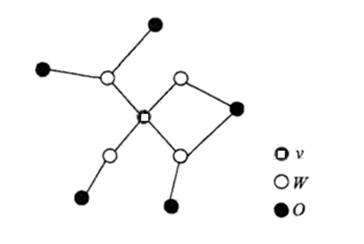全局马尔可夫性：设结点集合 A,B 是在无向图 G 中被结点集合 C 分开的任意结点集合，如图所示。结点集合 A,B 和 C 所对应的随机变量组分别是 YA,YB 和 YC。全局马尔可夫性是指给定随机变量组条件下随机变量组 YA 和 YB 是条件独立的，即
$P(Y_A,Y_B|Y_C) = P(Y_A|Y_C)P(Y_B|Y_C)$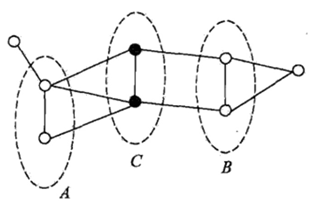概率无向图模型:
设有联合概率分布 P(Y) ，由无向图 G=(V,E) 表示，在图 G 中，结点表示随机变量，边表示随机变量之间的依赖关系。如果联合概率分布 P(Y) 满足成对、局部或全局马尔可夫性，就称此联合概率分布为概率无向图模型或马尔可夫随机场。
以上是概率无向图模型的定义，实际上，我们更关心的是如何求其联合概率分布。对给定的概率无向图模型，我们希望将整体的联合概率写成若干子联合概率的乘积的形式，也就是将联合概率进行因子分解，这样便于模型的学习与计算。事实上，概率无向图模型的最大特点就是易于因子分解。下面介绍这一结果。
概率无向图模型的因子分解
首先给出无向图中的团与最大团的定义，无向图 G 中任何两个结点均有边连接的结点子集称为团（clique)。若 C 是无向图 G 的一个团，并且不能再加进任何一个 G 的结点使其成为一个更大的团，则称此 C 为最大团（maximal clique)。
下图 (a) 表示由4个结点组成的无向图。图中由2个结点组成的团有5个:{$Y_1,Y_2$}, {$Y_2,Y_3$}, {$Y_3,Y_4$},{$Y_4,Y_2$},{$Y_1,Y_3$}.有2个最大团：{$Y_1,Y_2,Y_3$}，{$Y_2,Y_3,Y_4$},而 {$Y_1,Y_2,Y_3,Y_4$} 不是一个团，因为 Y1 和 Y4 没有边连接。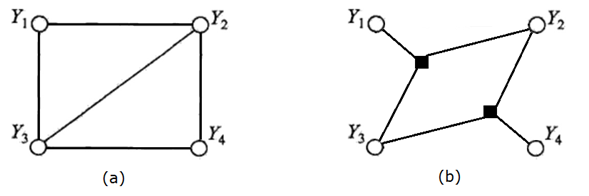将概率无向图模型的联合概率分布表示为其最大团上的随机变量的函数的乘积形式的操作，称为概率无向图模型的因子分解，譬如在解高次方程的时候，我们非常希望方程能够分解为多个低次方程的乘积。那么，对于概率分布函数而言，我们也希望能够这样做，即给定概率无向图模型，设无向图为 G ， C 为 G 上的最大团， YC  表示 C 对应的随机变量。那么概率无向图模型的联合概率分布 P(Y) 可分解为图中所有最大团 C 上的函数$\Psi_C(Y_C)$ 的乘积形式，分解后的因子图如 (b) 所示，每个黑色的正方形便代表一个函数，图中将无向图拆分为两个最大团上势函数的乘积，具体的拆分公式为：
$P(Y) = \frac{1}{Z} \prod_C \Psi_C(Y_C)$
其中，Z 是规范化因子（normalization factor)，形式如下：$Z = \sum_Y\prod_C \Psi_C(Y_C)$
规范化因子保证 P(Y) 构成一个概率分布。$\Psi_C(Y_C)$→R  称为势函数 (potential function)。这里要求势函数 $\Psi_C(Y_C)$ 是严格正的，通常定义为指数函数$\Psi_C(Y_C) = \exp \left \{-E(Y_C) \right \}$
总结一下,便得到 Hammersley-Clifford定理 ，概率无向图模型的联合概率分布可以表示为如下形式：
\begin{aligned} P(Y) &= \frac{1}{Z} \prod_C \Psi_C(Y_C) \\ Z &= \sum_Y\prod_C \Psi_C(Y_C) \end{aligned}
其中，C 是无向图的最大团， YC 是 C 的结点对应的随机变量， ΨC(YC)  是 C 上定义的严格正函数，乘积是在无向图所有的最大团上进行的。
条件随机场的模型表示
linear-chain 条件随机场
条件随机场（conditional random field）是给定随机变量 X 条件下，随机变量 Y 的马尔可夫随机场。本文主要介绍定义在线性链上的特殊的条件随机场，称为线性链条件随机场（linear-chain CRF)。线性链条件随机场可以用于机器学习里的标注问题。这时，在条件概率模型 P(Y|X) 中，Y 是输出变量，表示标记序列，也把标记序列称为状态序列（同 HMM 中的状态序列)；X 是输入变量，表示观测序。学习时，利用训练数据集通过极大似然估计或正则化的极大似然估计得到条件概率模型 $\hat{P}(Y|X)$ ；然后使用该模型预测即可。
CRF 的定义
设 X 与 Y 是随机变量，P(Y|X) 是在给定 X 的条件下 Y 的条件概率分布。若随机变量 Y 构成一个由无向图 G = (V,E) 表示的马尔可夫随机场，即：$P(Y_v|X,Y_w,w \ne v) =P(Y_v|X,Y_w,w \sim v)$
对任意结点 v 成立，则称条件概率分布 P(Y|X) 为条件随机场。式中 w∼v 表示在图 G = (V,E) 中与结点 v 有边连接的所有结点 w， w≠v 表示结点 v 以外的所有结点，$Y_u,Y_v,Y_w$ 为结点 u,v,w 对应的随机变量，从定义来看，左边到右边点的数量大大减小，w≠v 的点有 |V|−1 个，而 w∼v 就少了，其实就是说当前变量只跟与之相邻的变量有关系，而独立于没有直接连接的变量。
在定义中并没有要求 X 和 Y 具有相同的结构。现实中，一般假设 X 和 Y 有相同的图结构。本书主要考虑无向图为线性链的情况，即对于节点 1 到 n，边的情为： $E = \left \{ (i,i+1) \right \}_{i=1}^{n-1}$ ，在此情况下 $X =\left \{ X_i \right \}_{i=1}^{n} ,Y =\left \{ Y_i \right \}_{i=1}^{n}$，最大团是相邻两个结点的集合,下图即为 liner-chain CRF：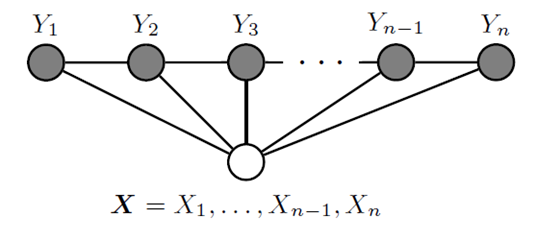线性链条件随机场的定义：设 $X =\left \{ X_i \right \}_{i=1}^{n} ,Y =\left \{ Y_i \right \}_{i=1}^{n}$均为线性链表示的随机变量序列，若在给定随机变量序列 X 的条件下，随机变量序列 Y 的条件概率分布 P(Y|X)构成条件随机场，即满足马尔可夫性
$P(Y_i | X,Y_1,…,Y_{i-1},Y_{i+1},…,Y_n) = P(Y_i | X,Y_{i-1},Y_{i+1})$
则称 P(Y|X) 为线性链条件随机场。注意当 i=1 或 i=n 时只考虑一侧，在标注问题中，X 表示输入观测序列，Y 表示对应的输出标记序列或状态序列。
CRF 的参数化形式
根据 Hammersley-Clifford 定理,可以给出线性链条件随机场 P(Y|X)的因子分解式，各因子是定义在相邻两个结点上的函数。在随机变量 X 取值为 x 的条件下，随机变量 Y 取值为 y 的条件概率具有如下形式：
$P(y|x) = \frac{1}{Z(x)}\exp \left \{ \sum_{i,k}\lambda_k t_k (y_{i-1},y_i,x,i)+ \sum_{i,l}\mu_l s_l(y_i,x,i) \right \}$
其中 Z(x) 为归一化项：$Z(x) = \sum_y \left \{ \sum_{i,k}\lambda_k t_k (y_{i-1},y_i,x,i)+ \sum_{i,l}\mu_l s_l(y_i,x,i) \right \}$
式中，$t_k$ 和 $s_l$是特征函数，$\lambda_k$ 和 $\mu_l$是对应的权值。 Z(x) 是规范化因子，求和是在所有可能的输出序列上进行的。以上两个式子是线性链条件随机场模型的基本形式，表示给定输入序列 x ,对输出序列 y 预测的条件概率。其中$t_k$ 是定义在边上的特征函数，称为转移特征（ t 是transition的缩写），依赖于当前和前一个位置， $s_l$是定义在结点上的特征函数，称为状态特征（s 是status的缩写），依赖于当前位置（无论哪种特征函数，都将当前可能的 yi 作为数）。$t_k$ 和 $s_l$ 都依赖于位置，是局部特征函数。通常，特征函数 $t_k$ 和 $s_l$ 取值为 1 或 0 ；当满足特征条件时取值为 1 ,否则为 0 。CRF 完全由特征函数和对应的权值 $\lambda_k$,$\mu_l$确定,线性链条件随机场也是对数线性模型（loglinear model)。
CRF 的简化形式
CRF 还可以由简化形式表示。注意到条件随机场式中同一特征在各个位置都有定义，可以对同一个特征在各个位置求和，将局部特征函数转化为一个全局特征函数，这样就可以将条件随机场写成权值向量和特征向量的内积形式，即条件随机场的简化形式，为简便起见，首先将转移特征和状态特征及其权值用统一的符号表示。设有 K1 个转移特征，K2 个状态特征,记f_k(y_{i-1},y_i,x,i) = \left \{ \begin{aligned} &t_k(y_{i-1},y_i,x,i), \ \ k = 1,2,...,K_1 \\ &s_t(y_i,x,i), \ \ \ \ \ \ \ \ \ \ k = K_1 + l ; l = 1,2,...,K_2 \end{aligned}\right.
上式其实是对特征函数进行编码，编号的前 K1 个属于转移特征，后 K2 个属于状态特征。记 K=K1+K2 ，编号统一了，后面就可以放到同一个矩阵里了。
然后，对转移与状态特征在各个位置 i 求和，记作
$f_k(y,x) = \sum_{i=1}^nf_k(y_{i-1},y_i,x,i), \ \ \ k = 1,2,…,K$
上式的特征函数虽然都写成接受 4 个参数的形式，但对状态特征函数而言，$y_{i-1}$是会被忽略掉的，用$w_k$ 表示特征$f_k(y,x)$的权值，即
w_k = \left \{ \begin{aligned} &\lambda_k, \ \ k = 1,2,...,K_1 \\ &\mu_l, \ \ k = K_1 + l ; l = 1,2,...,K_2 \end{aligned}\right.
于是，条件随机场可表示为：
\begin{aligned} P(y|x) &= \frac{1}{Z(x)} \exp \left \{ \sum_{k=1}^K w_k f_k(y,x) \right \}\\ Z(x) &= \sum_y \exp \left \{ \sum_{k=1}^Kw_kf_k(y,x)\right \} \end{aligned}
若 w 表示权值向量，即
$w= (w_1,w_2,…,w_K)^T$
以 F(y,x) 表示全局特征向量，即：$F(y,x) = \left \{ f_1(y,x), f_2(y,x),…,f_K(y,x) \right \}^T$
则条件随机场可以写成向量 w 与 F(y,x) 的内积的形式:
$P_w(y|x) = \frac{\exp\left \{w \cdot F(y,x)\right \} }{Z_w(x)}$
其中，
$Z_w(x) = \sum_y \exp \left \{ w \cdot F(y,x) \right \}$
条件随机场的矩阵形式
条件随机场还可以由矩阵表示。假设$P_w(y|x)$是由内积形式给出的线性链条件随机场，表示对给定观测序列 x ，相应的标记序列 y 的条件概率。引进特殊的起点和终点状态标记 $y_0 = start , y_{n+1} = stop$，这时$P_w(y|x)$可以通过矩阵形式表示。
对观测序列 x 的每一个位置 $i=1,2,…，n+1$，定义一个 m 阶矩阵（m 是标记 yi 取值的个数，因为 x 是给定的，\$ i-1 和位置 i 各有 m 种可能，所以是 m 阶矩阵）：
\begin{aligned} M_i(x) &= \left \{ M_i(y_{i-1},y_i|x)\right \} \\ M_i(y_{i-1},y_i|x)&= \exp \left \{ W_i(y_{i-1} ,y_i|x)\right \}\\ M_i(y_{i-1},y_i|x)&= \sum_{k=1}^Kw_k \cdot f_k(y_{i-1},y_i,x,i) \end{aligned}
其实矩阵定义了一个 状态 yi−1 的 m 种状态到 yi 的 m 种状态的转移的概率：
\begin{aligned} M_i(y_{i-1} ,y_i|x) &= \exp\left\{ \sum_k\lambda_kf_k(y_{i-1},y_i,x,i)\right\} \\ &=\exp\left\{ \sum_k\lambda_kt_k(y_{i-1},y_i,x,i) + \sum_l\mu_l s_l(y_i,x,i) \right\} \end{aligned}
举例来说，当 m = 3 时，除了 i =1 或者 i = n-1 ，每个矩阵 $M_i(x) \in\mathbb{R}^{3 \times 3}$， 如下图所示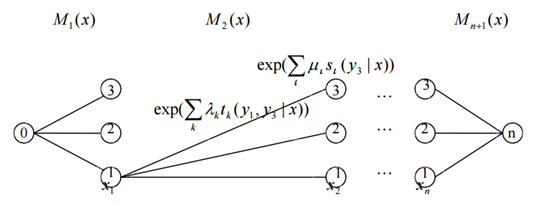矩阵的形式类似于 HMM 中的转移矩阵，代表了状态之间转移的概率，其形式是这样的:
\begin{aligned} M_1(x) &= \begin{bmatrix} M_1(y_0,y_1|x) & M_1(y_0,y_3|x) &M_1(y_0,y_3|x) \end{bmatrix} \\ \\ M_2(x) &=\begin{bmatrix} M_2(y_1,y_1|x) & M_2(y_1,y_2|x) & M_2(y_1,y_3|x)\\ M_2(y_2,y_1|x) & M_2(y_2,y_2|x) & M_2(y_2,y_3|x)\\ M_2(y_3,y_1|x) & M_2(y_3,y_2|x) & M_2(y_3,y_3|x) \end{bmatrix} \\ \\ M_i(x) \ &\mathbf{has \ the \ same \ form \ with} \ M_2(X), \ i = 3,...,n\\ \\ M_{n+1}(x) &=\begin{bmatrix} M_{n+1}(y_1,y_n|x)& \\ M_{n+1}(y_2,y_n|x) & \\ M_{n+1}(y_3,y_n|x)& \end{bmatrix} \\ \end{aligned}
这样，给定观测序列x,标记序列 y 的非规范化概率可以通过 n+1 个矩阵的乘积 $\prod_{i=1}^{n+1}M_i(y_{i-1},y_i|x)$表示，于是，条件概率 Pw(y|x) 是：
$P_w(y|x) = \frac{1}{Z_w(x)} \prod_{i=1}^{n+1} M_i(y_{i-1},y_i|x)$
其中，Zw(x) 为规范化因子，是 n+1 个矩阵的乘积的 (start,stop) 元素：
$Z_w(x) = \left \{M_1(x),M_2(x)…M_{n+1}(x) \right \} _{start}^{stop}$
注意，$y_0 = start$ 与$y_{n+1} = stop$ 表示开始状态与终止状态，规范化因子 Zw(x) 是以 start 为起点 stop 为终点通过状态的所有路径的非规范化概率 y1,y2,…,yn 之和。
这里的 M 矩阵像极了 HMM 中的转移概率矩阵，因为链式 CRF 中只有相邻两个节点间才有连接边。
条件随机场的概率计算问题
条件随机场的概率计算问题是给定条件随机场 P(Y|X) ，输入序列 x 和输出序列 y ,计算条件概率  $P(Y_{i-1} = y_{i-1}Y_i = y_i|x)$ ，$P(Y_i = y_i|x)$ 以及相应的数学期望的问题。为了方便起见，像 HMM 那样，引进前向-后向向量，递归地计算以上概率及期望值。这样的算法称为前向-后向算法。
前向-后向算法
对每个指标$i = 0,1,…,n+1$ ，定义前向向量 $a_i(x)$ ,对于起始状态 i=0：
a_0(y|x) = \left \{ \begin{aligned} &1, \ \ y = start \\ &0, \ \ else \end{aligned}\right.
对于之后的状态$i = 1,2,…,n+1$ ，递推公式为：
$a_i^T(y_i|x) = a^T_{i-1}(y_{i-1}|x)M_i(y_{i-1},y_i|x)$
这里$M_i(y_{i-1},y_i|x)$ 对应的是转移矩阵中的一列，转为向量形式可表示为
$a^T_i(x) = a^T_{i-1}(x)M_i(x)$
$a_i(y_i|x)$表示在位置 i 的标记是 yi 并且到位置 i 的前部分标记序列的非规范化概率，yi 可取的值有 m 个，所以 ai(x) 是 m 维列向量。
同样，对每个指标$i = 0,1,…,n+1$ ，定义后向向量$\beta_i(x)$:
\beta_{n+1}(y_{n+1}|x) = \left \{ \begin{aligned} &1, \ \ y_{n+1} = stop \\ &0, \ \ else \end{aligned}\right.
往前递推：
$\beta_i(y_i|x) = M_i(y_i,y_{i+1}|x)\beta_{i+1}(y_{i+1}|x)$
又可以表示为：
$\beta_i(x) = M_{i+1}(x) \beta_{i+1}(x)$
$\beta_i(y_i|x)$ 表示在位置 i 的标记为 $y_i$,并且从 i+1 到 n 的后部分标记序列的非规范化概率。
由前向-后向向量定义不难得到：
$Z(x) = a_n^T(x) \cdot \mathbf{1} = \mathbf{1}^T \cdot \beta_1(x)$
这里，1  是元素均为 1 的 m 维列向量。
概率计算
按照前向-后向向量的定义，很容易计算标记序列在位置 i 是标记 yi 的条件概率和在位置 i-1 与 i 是标记$y_{i-1}$ 和$y_{i}$的条件概率：
\begin{aligned} P(Y_i= y_i|x) &= \frac{a_i^T(y_i|x) \beta_i(y_i|x)}{Z(x)} \\ P(Y_{i-1} = y_{i-1} ,Y_i= y_i|x) &=\frac{a_{i-1}^T(y_{i-1}|x)M_i(y_{i-1},y_i|x)\beta_i(y_i|x)}{Z(x)} \end{aligned}
其中 $Z(x) = a_n^T(x) \cdot \mathbf{1}$
期望值的计算
利用前向-后向向量，可以计算特征函数关于联合分布 P(X,Y) 和条件分布 P(Y|X) 的数学期望。
特征函数 $\left \{ f_k \right \}_{k=1}^K$关于条件分布 P(Y|X) 的数学期望是
\begin{aligned} E_{p(Y|X)}[f_k] &= \sum_yP(y|x)f_k(y,x) \\ &=\sum_{i=1}^{n+1}\sum_{y_{i-1}\ y_i}f_k(y_{i-1},y_i,x,i) \frac{a_{i-1}^TM_i(y_{i-1},y_i|x)\beta_i(y_i|x)}{Z(x)} \end{aligned}
其中 $Z(x) = a_n^T(x) \cdot \mathbf{1}$
假设经验分布为 P˜(X) ，特征函数 $\left \{ f_k \right \}_{k=1}^K$ 关于联合分布 P(Y|X) 的数学期望是：
\begin{aligned} E_{P(X,Y)}[f_k] &= \sum_{x,y}P(x,y)\sum_{i=1}^{n+1}f_k(y_{i-1}.y_i,x,i) \\ &= \sum_x\widetilde{P}(x) \sum_yP(y|x)\sum_{i=1}^{n+1}f_k(y_{i-1,}y_ix,i) \\ &= \sum_x\widetilde{P}(x) \sum_{i=1}^{n+1} \sum_{y_{i-1} \ y_i}f_k(y_{i-1,}y_ix,i)\frac{a_{i-1}^T(y_{i-1}|x)M_i(y_{i-1},y_i|x) \beta_i(y_i|x)}{Z(x)} \end{aligned}